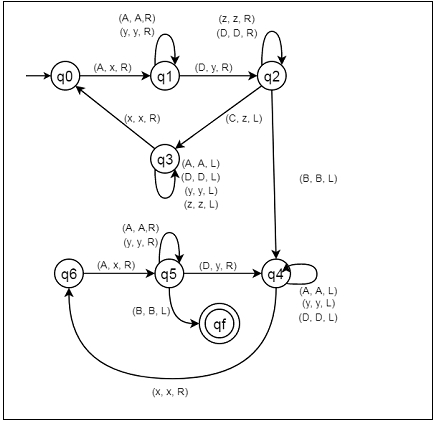# Construct a Turing machine for L = {aibjck | i>j>k; k ≥ 1}

C++Server Side ProgrammingProgramming

Here we will see how to make a Turing machine for language L = {AiBjCk | i > j > k; k ≥ 1}. So this represents a kind of language where we will use only three characters a, b and c. The w is a string. So if w = aaaaaabbbbccc, The Turing machine will accept it.

To solve this, we will use this approach. Firstly compare two elements by making A and D as a single element, after that comparing A and D if count of C is greater than |(A, D)|, then the string will not be accepted, if |D| > |A|, then this is not accepted, otherwise it will be accepted.

## State Transition Diagram